# Introduction

LeMans (Length-based Multispecies analysis by numerical simulation) is a length-structured fish community model, with the fish community being represented in terms of both species and length (Hall et al. 2006). By representing many processes, including fishing, natural mortality, and predation as functions of length, it is possible to reproduce many aspects of the community dynamics (including the tendency of diet to change with increasing predator size) with a relatively small number of parameters, and modest requirement for data in model set up. This makes the framework particularly suitable for use in data-limited fisheries.

Most assessment models have a single-species focus, which is appropriate for assessing the current state of the stock and making a very short term forecast, but in the longer-term, stock mortality and abundance will depend on its interactions with other parts of the food-web, and so in general any longer-term projections require the use of a multispecies model.

Assessment models are typically age-structured, i.e. they split the stock(s) being modelled into age classes (e.g. 1 year or 2 year old cod). This is the natural time frame for assimilating annual recruitment into a single-stock, but it is not useful for multispecies interactions, which are typically based upon the relative sizes of predator and prey, rather than being age-dependent. Consequently, LeMans represents stocks in terms of their length. This allows predation to emerge as a function of the relative lengths of predator and prey, and enables the observed shifts in diet as fish grow larger to emerge naturally rather than having to be ignored or hardwired (as for example happens in bulk biomass models such as surplus-production models or indeed Ecopath (Polovina 1984)). Size-structuring can be done either by weight (e.g. mizer; Scott, Blanchard, and Andersen (2014)), or length (e.g. FishSUMS; Speirs et al. (2010)). In LeMans we choose length because a) it is generally easier to measure than weight in the field (Conner, Matson, and Kelly 2017), and b) fisheries selectivity is normally characterised in terms of a relationship with length, which can more easily be related to the structure of fishing nets, and hence it is easier to relate to the parameterisation of mixed fisheries.

Unlike many size-based models, LeMans models predation in terms of a simple type II functional response rather than through the determination of encounter probabilities, and growth is assumed to follow the von Bertalanffy relationship rather than being food-limited, so the model would not be suitable for use in strongly food-limited ecosystems (e.g. the Baltic) unless modified. Where possible, generic relationships with length are applied to the key model processes, and this together with the assumption of von Bertalanffy growth makes the model data requirements relatively modest, facilitating its use in data-limited situations. Another key strength of the model is its ability to accommodate fleet dynamics (due to its length-based structure, simplicity, and speed of execution).

No model structure is perfect, and all involve making some trade-offs between comprehensiveness, run-time, flexibility and transparency, but LeMans is well suited for use whenever there is a need for multispecies or mixed fisheries analysis, but insufficient data to support the use of more complicated model, such as Ecopath or Atlantis (Fulton et al. 2011), provided that growth is not strongly food-limited.

# Setting up the model

## Dataset for the North Sea

In the LeMaRns package we supply data for the North Sea model (Thorpe et al. 2015). This includes the species parameters NS_par and the interaction matrix NS_tau.

NS_par
#>     species_names   Linf    W_a    W_b     k  Lmat           a
#> 1           Sprat  16.55 0.0059 3.1088 0.681 12.14 885.6667731
#> 2           Npout  23.75 0.0075 3.0244 0.849 17.21 225.0988055
#> 3         Sandeel  23.57 0.0049 2.7826 1.000 12.16 231.6879245
#> 4        Poor cod  23.00 0.0082 3.0865 0.520 15.00 254.2279144
#> 5  Long rough dab  25.00 0.0053 3.1434 0.340 15.00 185.3071561
#> 6             Dab  32.40 0.0159 2.8639 0.536 12.96  69.3184724
#> 7         Herring  33.35 0.0048 3.1984 0.606 23.39  62.1228287
#> 8  Horse mackerel  28.00 0.0316 2.6520 0.380 19.00 120.5695838
#> 9      Lemon sole  37.00 0.0123 2.9713 0.420 27.00  41.8978427
#> 10           Sole  46.41 0.0089 3.0172 0.284 20.96  17.7411663
#> 11       Mackerel  38.00 0.0052 3.1674 0.510 26.00  37.8676686
#> 12        Whiting  52.50 0.0099 2.9433 0.323 21.39  11.1149557
#> 13          Witch  44.00 0.0017 3.3887 0.200 29.00  21.7175060
#> 14        Gurnard  42.97 0.0160 2.8151 0.266 17.67  23.7587503
#> 15         Plaice  65.32 0.0125 2.9425 0.122 22.22   4.8535417
#> 16     Starry ray  66.00 0.0107 2.9400 0.230 46.00   4.6666095
#> 17        Haddock  70.65 0.0092 3.0126 0.271 26.91   3.6046815
#> 18     Cuckoo ray  92.00 0.0026 3.2169 0.110 59.00   1.3242529
#> 19       Monkfish 106.00 0.0297 2.8410 0.180 61.00   0.7738734
#> 20            Cod 149.87 0.0081 3.0502 0.216 54.39   0.2080939
#> 21         Saithe 118.94 0.0085 3.0242 0.175 48.61   0.4999956
#>               b
#> 1  8.038261e+03
#> 2  7.322485e+02
#> 3  8.249558e+04
#> 4  1.756348e+02
#> 5  1.848772e+02
#> 6  2.599582e+02
#> 7  7.507404e+03
#> 8  5.379469e+03
#> 9  2.518504e+01
#> 10 6.446078e+01
#> 11 2.872764e+03
#> 12 7.492533e+01
#> 13 8.760097e+00
#> 14 2.423226e+01
#> 15 9.366251e+01
#> 16 1.774109e+00
#> 17 6.599579e+01
#> 18 4.997712e-02
#> 19 2.105690e-01
#> 20 7.656718e-01
#> 21 1.066107e+01

NS_par contains several variables that are required to set up the model: Linf, the von-Bertalanffy asymptotic length of each species (cm); W_a and W_b, length-weight conversion parameters; k, the von-Bertalanffy growth parameter; Lmat the length at which 50% of the individuals are mature (cm); a, the density-independent part of the hockey-stick recruitment function and b, the density-dependent part of the hockey-stick recruitment function.

NS_tau is the interaction matrix for the species in the model. Each row of the matrix represents a prey species and each column represents a predator species. If element i,j is equal to 1 it means that the jth species predates on the ith species.

NS_other is other food for the North Sea.

## Setting the species-independent parameters

The LeMans model is based on a discrete time discrete length system where there are $$n_s$$ species and $$n_l$$ length classes with the $$l_j$$ being the lower length of the $$j$$th length class and $$l_{j+1}$$ being the upper limit. The mid point of the $$j$$th length class is $\begin{equation*} L_j=l_j+\frac{l_{j+1}-l_{j}}{2} \end{equation*}$ for $$j=1\ldots{}n_l$$.

To run LeMans model we must a number of species-independent parameters before it can be run. These parameters include: nfish, the number of species in the model; nsc, the number of length classes in the model and is set to 32 by default; and l_bound, u_bound and mid, which are the lower, upper and mid-points of the length classes respectively. The values of these parameters can be calculated by running:

nfish <- nrow(NS_par)
nsc <- 32
maxsize <- max(NS_par$Linf)*1.01 # the maximum size is 1% bigger than the largest Linf. l_bound <- seq(0, maxsize, maxsize/nsc); l_bound <- l_bound[-length(l_bound)] u_bound <- seq(maxsize/nsc, maxsize, maxsize/nsc) mid <- l_bound+(u_bound-l_bound)/2 phi_min is the time step of the model and can either be specified explicitly, with a default of 0.1, or calculated (see calc_phi in the next section). The LeMans model also has a numerical precision parameter eps, which is set to 1e-05 by default. ## Setting the species-specific parameters ### Size, growth and maturity In addition to the species-independent parameters described above, the LeMans model also requires a number of species-specific parameters. As a minimum the model requires: Linf <- NS_par$Linf # the von-Bertalanffy asymptotic length of each species (cm)
W_a <- NS_par$W_a # length-weight conversion parameter W_b <- NS_par$W_b # length-weight conversion parameter
k <- NS_par$k # the von-Bertalanffy growth parameter Lmat <- NS_par$Lmat # the length at which 50\% of individuals are mature (cm)

The length-weight equation is defined for species $$i$$ in length class $$j$$ as $\begin{equation*} W_{j,i}=a_iL_j^{b_i}. \end{equation*}$ In LeMaRns $$a_i$$ is represented by W_a and $$b_i$$ is represented by W_b. The LeMans model assumes that fish grow continuously throughout their lives, with the length of species $$i$$ at age $$t$$ being $\begin{equation*} L_{\infty,i}(1-e^{-k_i(t-t_{0,i})}), \end{equation*}$ where $$L_{\infty,i}$$ is the asymptotic length (cm) of the $$i$$th species, $$k_i$$ is the von Bertalanffy growth rate and $$t_{0,i}$$ is the theoretical age at which the $$i$$th species would be at length 0. In LeMaRns $$t_{0,i}$$ is not required, $$L_{\infty,i}$$ is represented by Linf and $$k_i$$ is represented by k.

The amount of time $$t$$ that species $$i$$ spends in length class $$j$$ is defined as: $\begin{equation} t_{j,i}=\frac{1}{k_i}\times\log\left(\frac{L_{\infty,i}-l_j}{L_{\infty,i}-l_{j+1}}\right). \end{equation}$

Assuming that individuals are evenly distributed across the length class, the proportion of individuals of species $$i$$ that leave length class $$j$$ due to growth over time $$\delta{}t$$ is $\begin{equation*} \phi_{j,i}=\frac{\delta{}t}{t_{j,i}}. \end{equation*}$

In LeMaRns $$\phi_{j,i}$$, $$\forall{}i,j$$, is represented by phi. In the example below we set phi_min equal to 0.1:

tmp <- calc_phi(k, Linf, nsc, nfish, u_bound, l_bound, calc_phi_min=FALSE, phi_min=0.1)
phi <- tmp$phi phi_min <- tmp$phi_min

The amount that a fish of species $$i$$ in length class $$j$$ will grow according to the von Bertalanffy growth curve in a time step, $$\delta{}t$$, is $\begin{equation*} \delta{}L_{j,i}=(L_{\infty,i} - L_{j,i})(1 - \exp(-k_i\delta{}t)). \end{equation*}$ This is then converted into weight increments, $$\delta{}W_{j,i}=a_i(\delta{}L_{j,i})^{b_i}$$, and then divided by the growth efficiency, $\begin{equation*} G_{j,i}=\left(1-\left(\frac{W_{j,i}}{W_{\infty,i}} \right)^\pi \right)\times{}g_0, \end{equation*}$ where $$W_{\infty,i}$$ is the weight of a fish of species $$i$$ of length $$L_{\infty,i}$$, $$\pi$$ is the rate at which the growth efficiency decays and $$g_0$$ is the growth efficiency of a fish of length zero. The amount of food required for the fish to grow according von Bertalanffy growth curve in a time step is then $\begin{equation*} I_{j,i}=\frac{\delta{}W_{j,i}}{G_{j,i}}. \end{equation*}$

This is calculated using the calc_ration_growthfac function. This calculates: ration, the food required (g) for a fish of average size in each length class to grow according von Bertalanffy growth curve in a time step; sc_Linf, the length class at which each species reaches Linf; wgt, a matrix with dimensions nsc and nfish representing the weight of each species in each length class; and g_eff, a matrix with dimensions nsc and nfish representing the growth efficiency of each species in each length class.

tmp <- calc_ration_growthfac(k, Linf, nsc, nfish, l_bound, u_bound, mid, W_a, W_b
, phi_min)
ration <- tmp$ration sc_Linf <- tmp$sc_Linf
wgt <- tmp$wgt g_eff <- tmp$g_eff

For species $$i$$ in length class $$j$$, the proportion of individuals that are mature is defined as: $\begin{equation*} M_{j,i}=\frac{1}{1+\exp\{-\kappa_i(L_j-L^{(mat)}_i)\}} \end{equation*}$ where $$L^{(mat)}_i$$ is the length at which 50% of individuals are mature and $$\kappa_i$$ is the rate of change from immaturity to maturity. In LeMaRns $$L^{(mat)}_i$$ is represented by Lmat and $$\kappa_i$$ is represented by kappa. In Thorpe et al. (2015), $$\kappa_i$$ is equal to 10 for all species. The proportion of individuals that are mature may thus be calculated by running:

mature <- calc_mature(Lmat, nfish, mid, kappa=rep(10, nfish), sc_Linf)

In the LeMans model, fish may feed on each other and on other food (g), which is referred to in LeMaRns as other.

other <- NS_other

### Recruitment

There are several different options for recruitment in LeMaRns. The recruitment function implemented in Hall et al. (2006) is the Ricker stock-recruitment function, which gives a dome-shaped relationship between spawning stock biomass (SSB) and the number of recruits and is defined, $\begin{equation*} R_i = \alpha_iS_ie^{-\beta_iS_i}, \end{equation*}$ where the species-specific parameters $$\alpha_i$$ and $$\beta_i$$ are the density-dependent and density-independent parameters respectively (Ogle 2016) and $$S_i$$ is the SSB in kilo-tonnes, i.e. $\begin{equation*} S_i=\frac{1}{10^{9}}\sum_{j=1}^{n_l}M_{j,i}N_{j,i}W_{j,i}, \end{equation*}$ where $$N_{j,i}$$ is the number of individuals of species $$i$$ in length class $$j$$.

In LeMaRns $$\alpha_i$$ is represented by $$a$$ and $$\beta_i$$ is represented by $$b$$. Below is an example with $$\alpha_i$$ set to 1.5 and $$\beta_i$$ set to 0.5: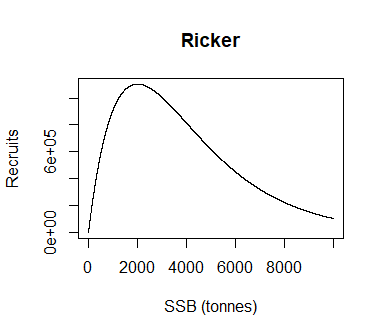It is also possible to implement the Beverton-Holt recruitment function: $\begin{equation*} R_i = \frac{\alpha_iS_i}{1 + \beta_iS_i}, \end{equation*}$ a linear recruitment function: $\begin{equation*} R_i = {\alpha_iS_i}, \end{equation*}$ a hockey-stick recruitment function: $\begin{equation*} R_i = \min({\alpha_iS_i}, R_{max,i}) \end{equation*}$ where $$R_{max,i}$$ is the maximum recruitment, or constant recruitment $$R_i$$. Below are examples of these recruitment functions: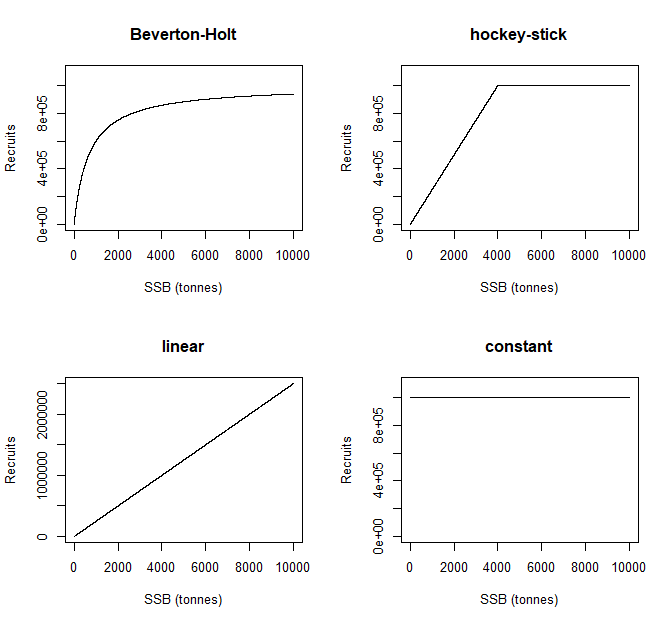To set up the hockey-stick function:

stored_rec_funs <- get_rec_fun(rep("hockey-stick", nfish))
recruit_params <- do.call("Map", c(c, list(a=NS_par$a, b=NS_par$b)))

For more details about recruitment see help(calc_recruits).

### Mortality

There are three types of mortality in the LeMans model: background mortality ($$M1$$), predation mortality ($$M2$$) and fishing mortality ($$F$$).

In LeMaRns, there are three different options for background mortality functions: std_RNM, constant and linear. std_RNM is the default option and gives a constant mortality rate, defined by Nmort, for the largest length classes and zero for the smallest. When using the std_RNM option, the parameter prop is used to define which length classes are considered to have a non-zero mortality rate. constant gives a constant mortality rate, defined by Nmort, for all length classes and linear gives M1=0 for the first length class, followed by a linear increase in $$M1$$ up to and including the sc_Linf-th length class where M1=Nmort.

Below gives examples of the different M1 functions for a species with an $$L_{\infty}$$ of 125: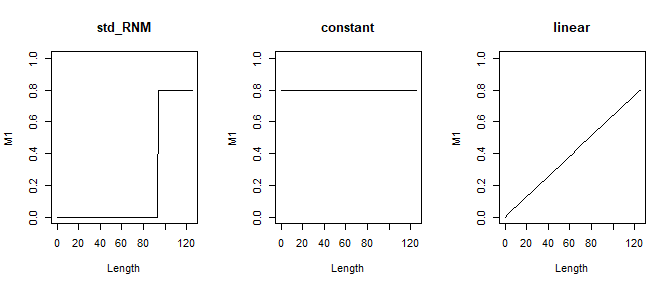To set up the std_RNM natural mortality function with a prop of 0.75 and an Nmort of 0.8:

M1 <- calc_M1(nsc, sc_Linf, phi_min,
natmort_opt=rep("std_RNM", length(sc_Linf)),
Nmort=rep(0.8, length(sc_Linf)),
prop=rep(0.75, length(sc_Linf)))

The species in the model interact with each other via predator-prey interactions. The prey weight preference $$w_p$$ for a predator of weight $$w$$ is defined as: $\begin{equation*} p(w, w_p)=\exp\left\{- \frac{\left(\log_{10}\left(\frac{w_p}{w}\right)-\mu_p\right)^2}{2\sigma_p} \right\}, \end{equation*}$ where $$\mu_p$$ is the preferred predator-prey mass ratio and $$\sigma_p$$ is the width of the weight preference function. In LeMaRns $$\mu_p$$ is represented by pred_mu, $$\sigma_p$$ is represented by pred_sigma and prey weight preferences are calculated by running:

prefs <- calc_prefs(pred_mu=-2.25, pred_sigma=0.5, wgt, sc_Linf)

The suitability of prey of weight $$w_p$$ and species $$j$$ for a predator of weight $$w$$ of species $$i$$ is defined as: $\begin{equation*} U(w,w_p,i,j)=p(w, w_p)\tau_{i,j}, \end{equation*}$ where $$\tau_{i,j}$$ is a value between 0 and 1 that represents the vulnerability of prey species $$j$$ to predator species $$i$$ (Hall et al. 2006). In LeMaRns prey suitability is calculated by running:

suit_M2 <- calc_suit_vect(nsc, nfish, sc_Linf, prefs, NS_tau)

This returns a list object of length nfish. Each element in the list is an array of dimensions nsc, nsc and nfish containing a value between 0 and 1 that represents a combination of prey preference and prey suitability for each species and length class, which is then used to determine predation mortality.

In the LeMans model, fishing mortality is dependent on effort and gear catchability. The instantaneous fishing mortality for species $$i$$ of in length class $$j$$ is $\begin{equation*} F_{j,i} = \sum_{k=1}^{H}e_kq_{i,k}(L_j), \end{equation*}$ where $$e_k$$ is the effort of the $$k$$th gear, for $$k=1\ldots{}H$$, and $$q_{i,k}(L_j)$$ is the catchability of a fish of length $$L_j$$ and species $$i$$ by gear $$k$$. Effort is added to the model during initialisation (see the next section for more details), whilst catchability is added when setting up the model. LeMaRns provides three in-built options for catchability curves: logistic, log_gaussian and knife-edge, but it also allows users to input their own catchability values using the custom input. The logistic curve is defined as: $\begin{equation*} q(L) = \frac{1}{1+\exp(-\eta(L-L_{50}))}, \end{equation*}$ where $$\eta$$ is the steepness of the slope of the catchability curve and $$L_{50}$$ is the length at 50% of the maximum catchability. This function gives a catchability of zero for the smallest length classes, a catchability of 1 for the largest length classes, and a smooth increase in catchability from zero to one around $$l_{50}$$. An example of a logistic catchability curve is shown below with an $$\eta$$ of 0.25 and an $$L_{50}$$ of 50: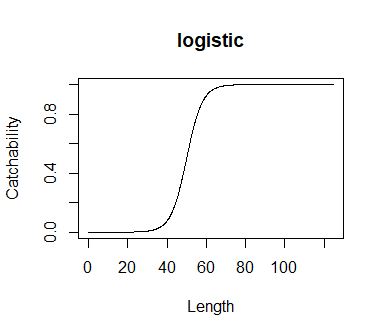The log_gaussian catchability function is defined as: $\begin{equation*} q(L) = \frac{1}{L\sigma\sqrt{2\pi}}\exp\left\{ -\frac{(\log(L)-\log(\mu_{50}))^2+\log(\mu_{50})-\sigma^2}{2\sigma^2} \right\} \end{equation*}$ where $$z$$ is …, $$\mu_{50}$$ is … and $$\sigma$$ is the standard deviation of the catchability curve. This function gives a dome-shaped relationship between length and catchability. An example of a log-gaussian catchability curve is shown below with a $$\mu_{50}$$ of 50 and a $$\sigma$$ of 1.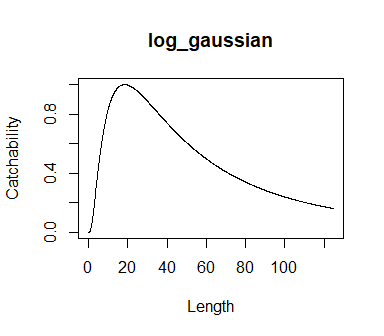The knife-edge function is $\begin{equation*} q(L)= \begin{cases} 1 & \quad \text{if } L\geq{}L_\mu\\ 0 & \quad \text{otherwise,} \end{cases} \end{equation*}$ where $$L_\mu$$ is the smallest length that is caught by the gear. An example of a knife-edge catchability curve is shown below with a $$L_\mu$$ of 50: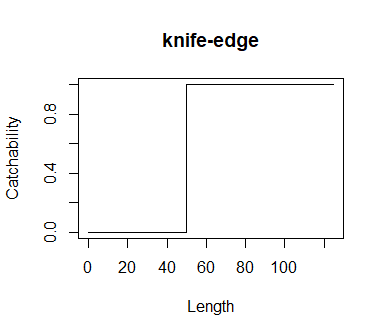We provide a case study that explores the use of multiple different gears at the end of the vignette, but for now we will set up a gear for each species that follows a logistic catchability curve with a $$L_{50}$$ at Lmat. In LeMaRns $$\eta$$ is represented by eta and $$L_{50}$$ is represented by L50:

Qs <- calc_Q(nsc=nsc, nfish=nfish, mid=mid, l_bound=l_bound, u_bound=u_bound,
species_names=NS_par$species_names,curve=rep("logistic", nfish), species=NS_par$species_names, max_catchability=rep(1, nfish),
gear_name=NS_par$species_names,eta=rep(0.25, nfish), L50=Lmat) ## LeMans_param objects A Lemans_param object can be used to store the model parameters (see help(Lemans_param) for further details). It is possible to create a Lemans_param object by running: NS_params <- new("LeMans_param", nsc=nsc, nfish=nfish, phi_min=phi_min, l_bound=l_bound, u_bound=u_bound, mid=mid, species_names=NS_par$species_names,
Linf=Linf,
W_a=W_a,
W_b=W_b,
k=k,
Lmat=Lmat,
mature=mature,
sc_Linf=sc_Linf,
wgt=wgt,
phi=phi,
ration=ration,
other=other,
M1=M1,
suit_M2=suit_M2,
Qs=Qs,
stored_rec_funs=stored_rec_funs,
eps=1e-05,
recruit_params=recruit_params)

Alternatively a Lemans_param object can be created using the LeMansParam function. This function can be used in several ways. For example, the parameters can be given individually:

NS_params <- LeMansParam(species_names=NS_par$species_names, Linf=Linf, k=k, W_a=W_a, W_b=W_b, Lmat=Lmat, tau=NS_tau, recruit_params=list(a=NS_par$a, b=NS_par$b), eta=rep(0.25, 21), L50=Lmat) the species parameters can be provided as a data.frame (referred to as df): NS_params <- LeMansParam(NS_par, tau=NS_tau, eta=rep(0.25, 21), L50=NS_par$Lmat, other=NS_other)

and/or the gear types can be provided as a data.frame (referred to as gdf; see the gear examples below). As a minimum the species data frame must contain Linf, k, W_a, W_b and Lmat. It is also possible to include species_names, kappa, rec_fun, natmort_opt, Nmort and prop. Any additional variables are assumed to be recruitment parameters and a warning is shown to highlight this, i.e.

#> Warning in .local(df, gdf, ...): The following columns of df do not match any of the species arguments and were therefore added to recruit_params:
#> a, b

# Running the model

The LeMans model works by projecting the number of individuals in each length class for each species forward in time. N is therefore a nsc by nfish matrix where N[j,i] is the number of individuals of species i that are in length class j. We will refer to $$N_{j,i,t}$$ as the number of individuals of species $$i$$ in length class $$j$$ and time $$t$$.

## Initialising the model

Given all of the aforementioned parameters, the minimum requirement for the model to be run is an initial matrix N. N can be calculated using an intercept of e.g. 1e10 and a slope of e.g. -5 by running:

N <- get_N0(nsc, nfish, mid, wgt, sc_Linf, intercept=1e10, slope=-5)

In this function the total number of individuals in the community in each length class is equal to intercept*mid^slope. Within each length class, the number of individuals of each species is determined using the proportion of each species’ biomass that is found in that length class. We can now plot the initial size spectrum of the model: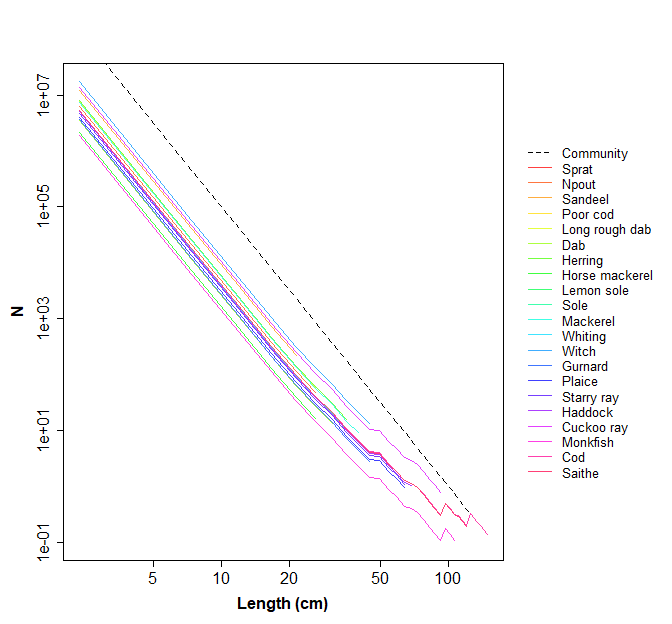The fishing mortality, Fs, must also be defined. To do this we need to define the effort for each of the gears Qs. In this example we will set the effort to be 0.5 for all species:

effort <- rep(0.5, dim(Qs))
Fs <- matrix(0, nsc, nfish)
for (j in 1:length(effort)) {
Fs <- Fs+effort[j]*Qs[,,j]
}

## The model run

The model is run for many time steps, with the length of each time step being equal to phi_min. At each time step three processes occur: recruitment, mortality and growth.

### Recruitment

In Thorpe et al. (2015) and Hall et al. (2006) recruitment only occurs in the first time step of a new year. The number of individuals after the recruitment phase of the model $$N'_{j,i,t}$$ is thus defined as $$N'_{j,i,t}=N_{j,i,t-1}$$ for $$j=2,\ldots,n_l$$ and $\begin{equation*} N'_{1,i,t} = N_{1,i,t-1}+R_{i,t}, \end{equation*}$ where $$R_{i,t}$$ is the number of recruits of species $$i$$ at time $$t$$. When the recruitment phase is implemented in LeMaRns the SSB is first calculated in grams and then the number of recruits is calculated:

SSB <- calc_SSB(mature, N, wgt)
R <- calc_recruits(SSB, stored_rec_funs, recruit_params)
N[1, ] <- N[1,]+R

### Mortality

The number of individuals after the mortality phase of the model $$N''_{j,i,t}$$ is defined as: $\begin{equation*} N''_{j,i,t}=N'_{j,i,t}\exp(-(M1_{j,i}+M2_{j,i,t}+F_{j,i,t})\delta{}t). \end{equation*}$ where $$M1$$ is the background mortality, $$M2$$ is the predation mortality and $$F$$ is the fishing mortality (represented by Fs in LeMaRns). The predation mortality of a fish of species $$i$$ in length class $$j$$ is $\begin{equation*} M2_{j,i,t}=\sum_{m=1}^{n_s}\sum_{n=1}^{n_l}I_{j,i}N'_{j,i,t}\frac{U(W_{n,m},W_{j,i},m,i)}{\sum_{k=1}^{n_s}\sum_{l=1}^{n_l}U(W_{n,m},W_{l,k},m,k)W_{l,k}N_{l,k} + o} \end{equation*}$ where $$o$$ is other food.

The catch of species $$i$$ in length class $$j$$ is $\begin{equation*} C_{j,i,t}= N'_{j,i,t}(1-\exp(-F_{j,i,t}\delta{}t)). \end{equation*}$

In LeMaRns, M2 is calculated and then added to Fs, M1 and a numerical stability parameter known as eps. Total mortality Z and the Catch for each species and length class (g) is calculated by running:

M2 <- calc_M2(N, ration, wgt, nfish, nsc, other, sc_Linf, suit_M2)
Z <- Fs+M1+M2+1e-05
Catch <- (Fs/Z)*N*(1-exp(-Z))*wgt
N <- N*exp(-Z)

### Growth

The number of individuals after the growth phase $$N_{j,i,t}$$ is defined as: $\begin{equation*} N_{j,i,t}= \begin{cases} N''_{j,i,t}(1-\phi_{j,i}) & \quad \text{if } j=1\\ N''_{j,i,t}(1-\phi_{j,i}) + N''_{j-1,i,t}\phi_{j-1,i} & \quad \text{otherwise} \end{cases} \end{equation*}$ In LeMaRns, growth is calculated by running:

N <- calc_growth(N, phi, nfish, nsc)

## run_LeMans function

Alternatively, the run_LeMans function can be used to run a dynamic version of the model over a given number of years (as defined by years).

There are several ways to use the run_LeMans function. All of the parameters can be added to the function explicitly. To do this, we first need to define the initial population and set the correct number of time steps. Below we run the model for 50 years:

N0 <- get_N0(nsc, nfish, mid, wgt, sc_Linf, intercept=1e10, slope=-5) # initialise N
years <- 50 # run for 10 years
tot_time <- years*phi_min # calculate the total number of time steps

We then need to define the fishing mortality. In the example below we fish for the duration of the model run with $$e_k$$ equal to 0.5 for all gears:

effort <- matrix(0.5, tot_time, dim(Qs))
# Calculate F
Fs <- array(0, dim=c(nsc, nfish, tot_time))
for (j in 1:ncol(effort)) {
for (ts in 1:tot_time) {
Fs[,,ts] <- Fs[,,ts]+effort[ts, j]*Qs[,,j]
}
}

We are then in a position to run the model:

model_run <- run_LeMans(N0=N0, tot_time=tot_time, Fs=Fs, nsc=nsc, nfish=nfish, phi_min=phi_min,
mature=mature, sc_Linf=sc_Linf, wgt=wgt, phi=phi, ration=ration,
other=other, M1=M1, suit_M2=suit_M2, stored_rec_funs=stored_rec_funs,
recruit_params=recruit_params, eps=1e-05)

Alternatively we can pass a LeMans_param object to the run_LeMans function:

model_run <- run_LeMans(NS_params, N0=N0, Fs=Fs, tot_time=tot_time)

Another alternative is to use the run_LeMans function to initialise the model as well. To do this, effort must be defined as a years by dim(Qs) matrix where the fishing effort remains the same for a whole year:

effort_mat <- matrix(0.5, years, dim(Qs))
model_run <- run_LeMans(NS_params, years=50, effort=effort_mat)

# Exploring the outputs

The run_LeMans function returns a LeMans_output object. A LeMans_output object has four slots: N, an array with dimensions nsc, nfish and tot_time representing the number of individuals in each length class at each time step of the model; Catch, an array with dimensions nsc, nfish and tot_time representing the biomass (g) of each species that is caught in each length class at each time step of the model; M2, an array with dimensions nsc, nfish and tot_time representing the predation mortality of each species in each length class at each time step of the model; and R, a matrix with dimensions tot_time and nfish representing the number of recruits of each species at each time step of the model.

The LeMaRns package includes a number of in-built functions that enable users to explore the outputs of a model run in more detail. These functions can be used to calculate and plot community and species-specific biomass and SSB, as well as several ecosystem indicators including the Large Fish Indicator (LFI), Mean Maximum Length (MML), Typical Length (TyL) and Length Quantiles (LQ). There are also functions to calculate Catch Per Unit Effort (CPUE) and Catch Per Gear (CPG).

## Biomass

The biomass of each species at time $$t$$ is defined as: $\begin{equation*} B_{i,t}=\sum_{j=1}^{n_l}N_{j,i,t}W_{j,i}. \end{equation*}$

In LeMaRns the biomass of each species can be calculated using the get_biomass function. There are several ways in which this function can be used. For example, users can pass N and wgt to the function directly:

biomass <- get_biomass(N=N, wgt=wgt)

or they can provide both LeMans_param and a LeMans_output objects:

biomass <- get_biomass(inputs=NS_params, outputs=model_run)

Options also exist that allow users to specify the species and time_steps they would like the to calculate the biomass for. species can be specified either as a numeric vector:

biomass <- get_biomass(inputs=NS_params, outputs=model_run, time_steps=1:500, species=1:4)

or as a character vector:

biomass <- get_biomass(inputs=NS_params, outputs=model_run, time_steps=1:500,
species=c("Herring", "Whiting", "Haddock", "Cod"))

If more than one species is provided, the get_biomass() function returns a matrix with dimensions length(time_steps) by length(species) where the $${i,j}$$th element is the biomass (g) of the $$j$$th species in the $$i$$th time_steps. If only one species is provided, the function returns a numeric vector of length length(time_steps).

A similar set of methods can be used to plot the biomass of each species using the plot_biomass function. For example, running either:

plot_biomass(N=N, wgt=wgt, time_steps=1:500, species=1:4)

or

plot_biomass(inputs=NS_params, outputs=model_run, time_steps=1:500, species=1:21)

returns the following plot: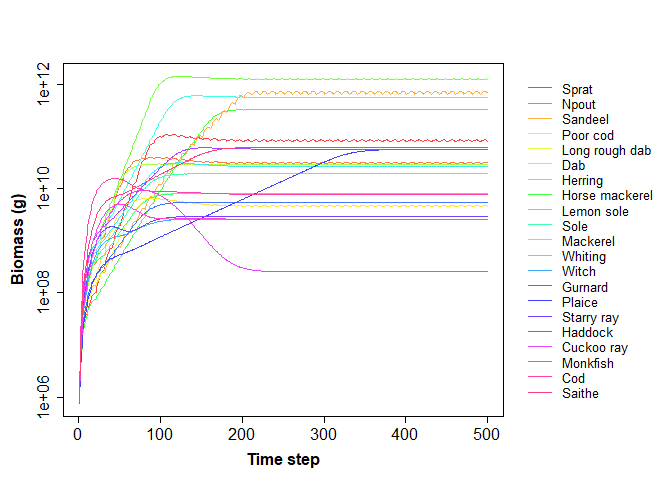Alternatively, users may pass the outputs of get_biomass to the plot_biomass function directly as shown below:

biomass <- get_biomass(inputs=NS_params, outputs=model_run)
plot_biomass(biomass=biomass, time_steps=1:500, species=1:4)

The full_plot_only option allows users to specify whether individual plots should also be produced for each species. For example, the following plots are returned by setting full_plot_only to FALSE:

plot_biomass(inputs=NS_params, outputs=model_run, time_steps=1:500, species=1:4,
full_plot_only=FALSE)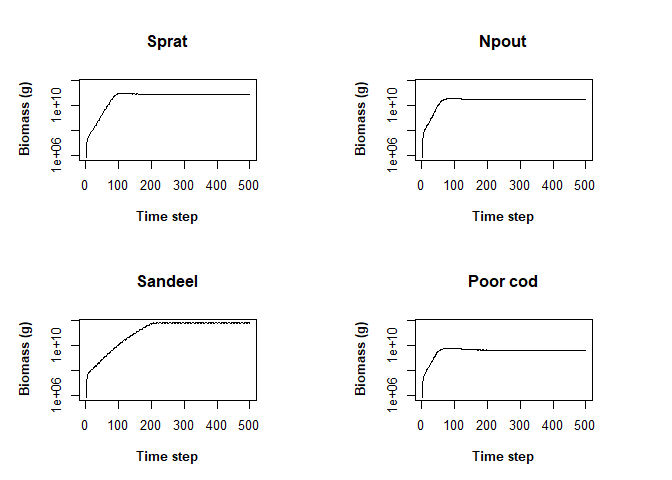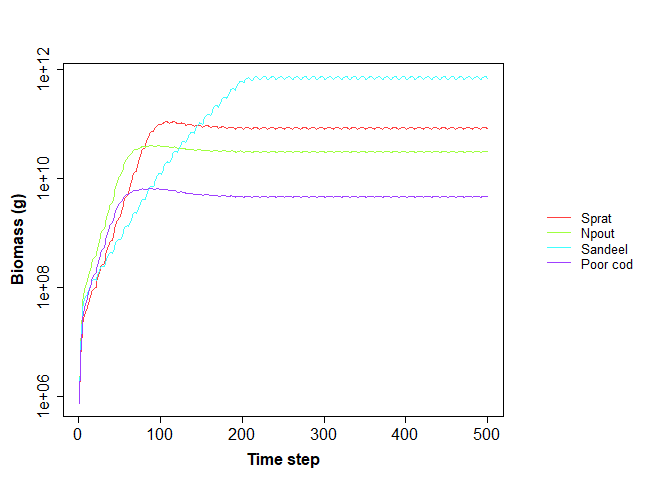Note that if a LeMans_param object is passed to the plot_biomass function, the plot will be labelled with the correct species names. However, if a LeMans_param object is not provided, the species will be labelled as “Species 1”, “Species 2” etc. unless users specify species_names directly.

## Spawning Stock Biomass (SSB)

The SSB of each species at time $$t$$ is defined as: $\begin{equation*} SSB_{i,t}=\sum_{j=1}^{n_l}M_{j,i}N_{j,i,t}W_{j,i} \end{equation*}$ where $$M_{j,i}$$ is the proportion of mature individuals of species $$i$$ in length class $$j$$.

In LeMaRns the SSB of each species can be calculated using the get_SSB function and plotted using the plot_SSB function, both of which work in exactly the same way as get_biomass and plot_biomass as described above. For example, species-specific SSB may be calculated and plotted by running:

SSB <- get_SSB(inputs=NS_params, outputs=model_run, time_steps=1:500, species=1:21)
plot_SSB(SSB=SSB, time_steps=1:500, species=1:21, species_names=NS_params@species_names)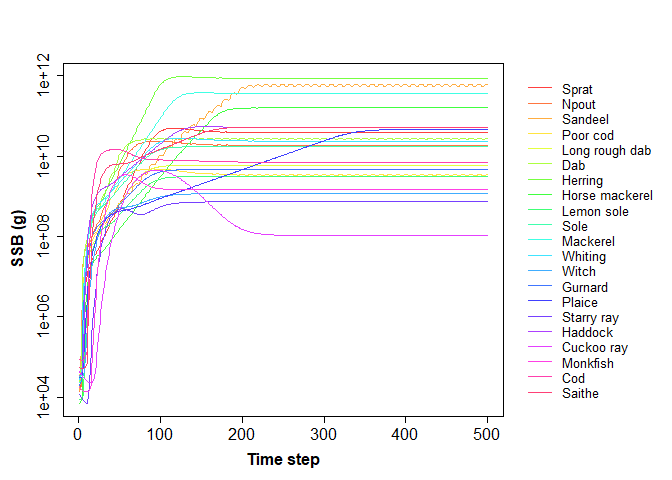## Indicators

As previously mentioned, LeMaRns also includes in-built functions that can be used to quantify and plot several proposed indicators of ecosystem health, including the Large Fish Indicator (LFI), Mean Maximum Length (MML), Typical Length (TyL) and Length Quantiles (LQ).

The LFI represents the proportion of the biomass with a length larger than $$L_{LFI}$$ (cm) at time $$t$$: $\begin{equation*} LFI_t=\frac{\sum_{i=1}^{n_s}\sum_{j=1}^{n_l}N_{j,i,t}W_{j,i}I(L_{i}\geq L_{LFI})}{\sum_{i=1}^{n_s}B_{i,t}}, \end{equation*}$ where $$I$$ is an indicator function that takes a value of one if $$L_i\geq L_{LFI}$$ is TRUE and zero if it is FALSE. In LeMaRns $$L_{LFI}$$ is represented by length_LFI and is set to 40cm by default, but it can take any length and can also take on multiple values if required (see below for example).

MML represents the biomass-weighted mean of $$L_{\infty,i}$$ at time $$t$$: $\begin{equation*} MML_t=\frac{\sum_{i=1}^{n_s} B_{i,t} L_{\infty,i}}{\sum_{i=1}^{n_s} B_{i,t}} \end{equation*}$ where $$L_{\infty,i}$$ represents the asymptotic length for the $$i$$th species in a community of $$m$$ species and is denoted as Linf in LeMaRns.

TyL represents the biomass-weighted geometric mean length of the community at time $$t$$: $\begin{equation*} TyL_t=\exp \left[\frac{\sum_{i=1}^{n_s} N_{j,i,t}W_{j,i} \log(L_j)}{\sum_{i=1}^{n_s} B_{i,t}}\right] \end{equation*}$

LQ represents the length $$L_{p,t}$$ at which biomass exceeds a given proportion $$p$$ of the total biomass at time $$t$$. This is calculated by solving: $\begin{equation*} p=\frac{\sum_{i=1}^{n_s}\sum_{j=1}^{n_l}N_{j,i,t}W_{j,i}I(L_{i}\leq L_{p,t})}{\sum_{i=1}^{n_s}B_{i,t}} \end{equation*}$ for $$L_{p,t}$$. In LeMaRns $$p$$ is represented by prob and is set to 0.5 by default, but it can take any value between 0 and 1 and can also take multiple values if required (see below for example).

The LFI, MML, TyL and LQ can be calculated using the get_LFI, get_MML, get_TyL and get_LQ functions respectively, which again work in a similar way to the get_biomass function. For example, each indicator may be calculated by running:

LFI <- get_LFI(inputs=NS_params, outputs=model_run, time_steps=1:500, species=1:21,
length_LFI=c(30, 40))
MML <- get_MML(inputs=NS_params, outputs=model_run, time_steps=1:500, species=1:21)
TyL <- get_TyL(inputs=NS_params, outputs=model_run, time_steps=1:500, species=1:21)
LQ <- get_LQ(inputs=NS_params, outputs=model_run, time_steps=1:500, species=1:21,
prob=c(0.5, 0.95))

If more than one length_LFI is specified, the get_LFI function will return a matrix with dimensions length(time_steps) by length(length_LFI) where the $${i,j}$$th element is the LFI given by the $$j$$th length_LFI in the $$i$$th time_steps. If only one species is provided, the function will return a numeric vector of length length(time_steps). The same is also true when more than one prob is passed to the get_LQ function. Both the get_MML and get_TyL functions always return a numeric vector of length length(time_steps).

The LFI, MML, TyL and LQ can be plotted using the plot_LFI, plot_MML, plot_TyL and plot_LQ functions respectively. Users can either provide LeMans_param and LeMans_output objects to the plot functions:

plot_LFI(inputs=NS_params, outputs=model_run, time_steps=1:500, species=1:21,
length_LFI=c(30, 40))
plot_MML(inputs=NS_params, outputs=model_run, time_steps=1:500, species=1:21)
plot_TyL(inputs=NS_params, outputs=model_run, time_steps=1:500, species=1:21)
plot_LQ(inputs=NS_params, outputs=model_run, time_steps=1:500, species=1:21,
prob=c(0.5, 0.95))

or they can provide the outputs of get_LFI, get_MML, get_TyL and get_LQ to the plot functions directly:

plot_LFI(LFI, length_LFI=c(30, 40))
plot_MML(MML)
plot_TyL(TyL)
plot_LQ(LQ, prob=c(0.5, 0.95))

In both cases, the following plots will be returned: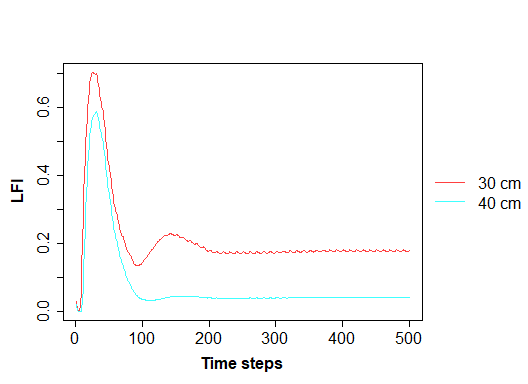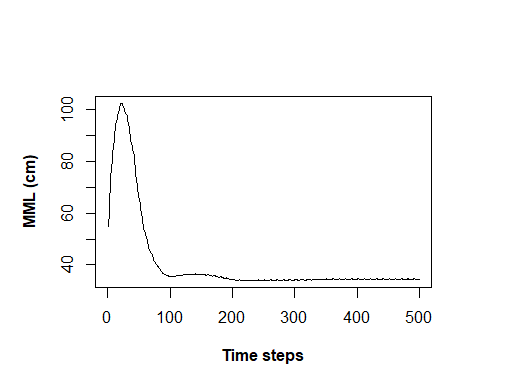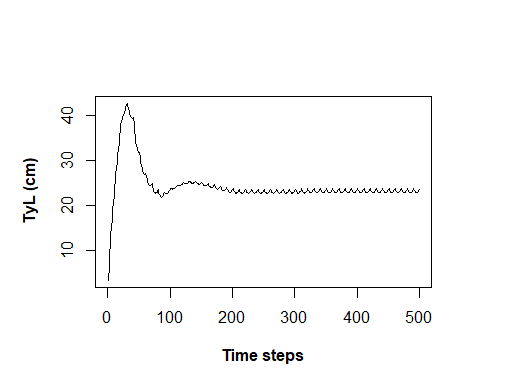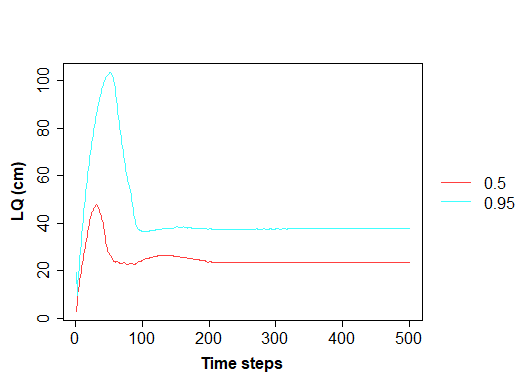LeMaRns also includes the get_indicators and plot_indicators functions, which can be used to calculate and plot all four indicators using a single function call. For example, the indicators can be calculated by running:

indicators <- get_indicators(inputs=NS_params, outputs=model_run, time_steps=1:500,
species=1:21,length_LFI=c(30, 40), prob=c(0.5, 0.95))

which returns a list object with the names LFI, MML, TYL and LQ.

To plot the indicators, users can again provide either LeMans_param and LeMans_output objects:

plot_indicators(inputs=NS_params, outputs=model_run, time_steps=1:500, species=1:21,
length_LFI=c(30, 40), prob=c(0.5, 0.95))

or they can provide the output of get_indicators to the plot function directly:

plot_indicators(LFI=indicators[['LFI']], MML=indicators[['MML']],
TyL=indicators[['TyL']], LQ=indicators[['LQ']],
time_steps=1:500, species=1:21,
length_LFI=c(30, 40), prob=c(0.5, 0.95))

In both cases, the following plot will be returned: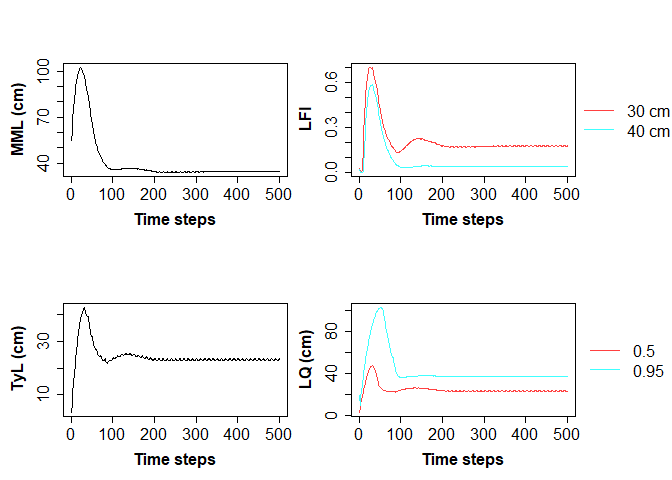## Catch

Finally, LeMaRns includes in-built functions that can be used to explore catch. Catch Per Unit Effort (CPUE) and Catch Per Gear (CPG) can be calculated using the get_CPUE() and get_CPG() functions in the same way as described previously:

CPUE <- get_CPUE(inputs=NS_params, outputs=model_run, time_steps=1:500, species=1:21)
CPG <- get_CPG(inputs=NS_params, outputs=model_run, time_steps=1:500, species=1:21)

# Case Study

In this section we give two examples of mixed fisheries and an example of finding long-term fishing targets. In the first we examine the effect on the fish species of various fishing efforts on different fleets and in the second demonstrate an example with dynamic fishing mortality. The final example calculates a Nash equilibrium.

Please note that the parameters in the example dataset in LeMaRns have not been calibrated to observations. Therefore the case studies are demonstrations of the package and the results should not be interpreted. For methods on calibrating these kinds of models see Thorpe et al. (2015) or Spence, Blackwell, and Blanchard (2016).

## Fishing fleets

In this case study we explore the mixed fishery example described in Thorpe et al. (2016), which involves four different fishing fleets. Here, we use idealised fleets, i.e. a single fish is caught by only one gear type. The data frame NS_mixed_fish contains the information on which fleet catches which species:

NS_mixed_fish
#>     catch_species    curve  gear_name max_catchability
#> 1           Sprat logistic Industrial            1.060
#> 2           Npout logistic Industrial            0.477
#> 3         Sandeel logistic Industrial            0.573
#> 4        Poor cod logistic      Otter            0.548
#> 5  Long rough dab logistic       Beam            0.548
#> 6             Dab logistic       Beam            0.548
#> 7         Herring logistic    Pelagic            0.247
#> 8  Horse mackerel logistic    Pelagic            0.627
#> 9      Lemon sole logistic       Beam            0.548
#> 10           Sole logistic       Beam            0.534
#> 11       Mackerel logistic    Pelagic            0.627
#> 12        Whiting logistic      Otter            0.365
#> 13          Witch logistic       Beam            0.548
#> 14        Gurnard logistic      Otter            0.548
#> 15         Plaice logistic       Beam            0.548
#> 16     Starry ray logistic       Beam            0.548
#> 17        Haddock logistic      Otter            0.577
#> 18     Cuckoo ray logistic       Beam            0.548
#> 19       Monkfish logistic      Otter            0.548
#> 20            Cod logistic      Otter            0.882
#> 21         Saithe logistic      Otter            0.377

In this example, the selectivity of each species follows the logistic curve and requires the parameters NS_eta and NS_L50, which are provided as part of LeMaRns.

We first set up the LeMans_params object:

NS_params <- LeMansParam(df=NS_par, gdf = NS_mixed_fish, tau=NS_tau, eta=NS_eta, L50=NS_L50, other=NS_other)
#> Warning in .local(df, gdf, ...): The following columns of df do not match any of the species arguments and were therefore added to recruit_params:
#> a, b

Initially we will examine the virgin biomass by running the model to equilibrium with no fishing. To do this, we set up effort and then run the model for 50 years:

effort_mat <- matrix(0, 50, dim(NS_params@Qs))
colnames(effort_mat) <- c("Industrial", "Otter", "Beam", "Pelagic")
model_run <- run_LeMans(NS_params, years=50, effort=effort_mat)

We plot Spawning Stock Biomass (SSB) to ensure it has reached equilibrium:

plot_SSB(inputs=NS_params, outputs=model_run, full_plot_only=TRUE)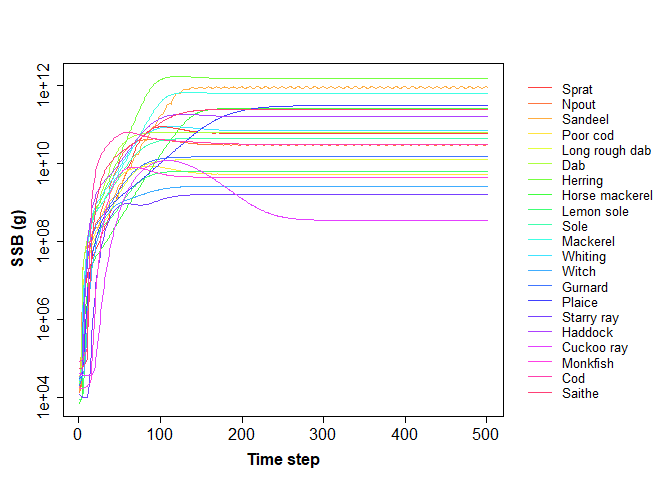and then check the indicators:

plot_indicators(inputs=NS_params, outputs=model_run)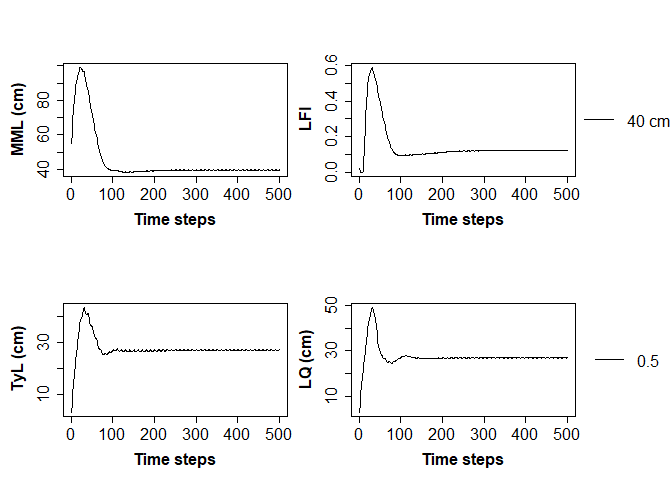We take the mean of the final year of the simulation to calculate the virgin SSB:

v_SSB <- colMeans(get_SSB(inputs=NS_params, outputs=model_run, time_steps=492:501))/1e6

In Thorpe et al. (2016) a factorial design was used to explore the effects of changing the fishing effort of different fleets. In this example we allow each of the four fleets to fish at one of five effort levels and set up a factorial design:

ef_lvl <- c(0, 0.5, 1, 1.5, 2)
efs <- expand.grid(Industrial=ef_lvl, Otter=ef_lvl, Beam=ef_lvl, Pelagic=ef_lvl)

We run the LeMans model for 50 years from the final state of the model in the model_run above. For efficiency we create a function that takes in the factorial design and then runs the LeMans model:

run_the_model<- function(ef){
effort_mat <- matrix(ef, 50, dim(NS_params@Qs), byrow=T)
colnames(effort_mat) <- c("Industrial", "Otter", "Beam", "Pelagic")
model_run <- run_LeMans(params=NS_params, N0=model_run@N[,,501],
years=50, effort=effort_mat)
return(model_run)
}

We then run the model over the factorial design (warning this may take a few minutes):

sce <- apply(efs, 1, run_the_model)

We then calculate the Large Fish Indicator (LFI), Typical Length (TyL) and Mean Maximum Length (MML) for all of the model runs:

LFI <- unlist(lapply(lapply(sce, FUN=get_LFI, time_steps=492:501, inputs=NS_params),
mean))
TyL <- unlist(lapply(lapply(sce, FUN=get_TyL, time_steps=492:501, inputs=NS_params),
mean))
MML <- unlist(lapply(lapply(sce, FUN=get_MML, time_steps=492:501, inputs=NS_params),
mean))

We can now investigate the effect of changing the effort of each of the fishing gears on the above indicators using box-plots:

par(mfrow=c(2, 2))
boxplot(LFI~efs[, 1], main="Industrial", xlab="Effort", ylab="LFI")
boxplot(LFI~efs[, 2], main="Otter", xlab="Effort", ylab="LFI")
boxplot(LFI~efs[, 3], main="Beam", xlab="Effort", ylab="LFI")
boxplot(LFI~efs[, 4], main="Pelagic", xlab="Effort", ylab="LFI")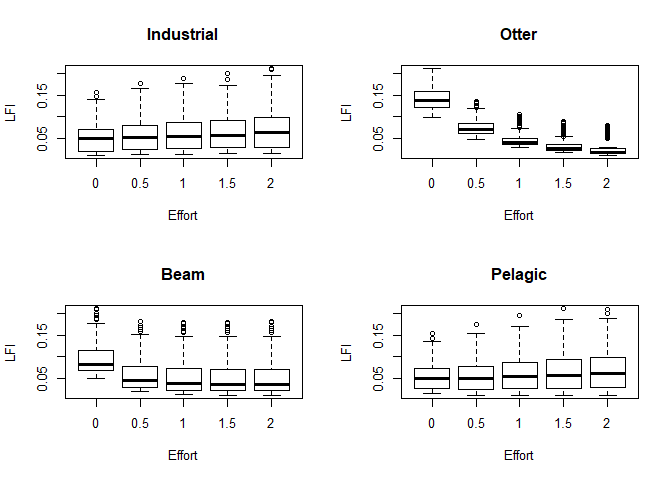par(mfrow=c(2, 2))
boxplot(TyL~efs[, 1], main="Industrial", xlab="Effort", ylab="TyL")
boxplot(TyL~efs[, 2], main="Otter", xlab="Effort", ylab="TyL")
boxplot(TyL~efs[, 3], main="Beam", xlab="Effort", ylab="TyL")
boxplot(TyL~efs[, 4], main="Pelagic", xlab="Effort", ylab="TyL")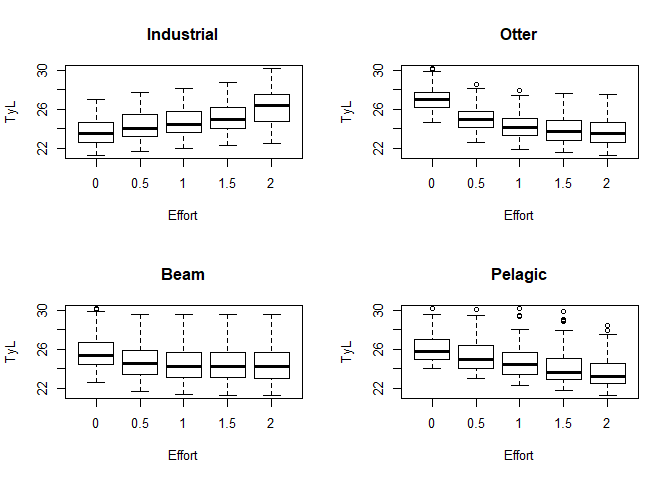par(mfrow=c(2, 2))
boxplot(MML~efs[, 1], main="Industrial", xlab="Effort", ylab="MML")
boxplot(MML~efs[, 2], main="Otter", xlab="Effort", ylab="MML")
boxplot(MML~efs[, 3], main="Beam", xlab="Effort", ylab="MML")
boxplot(MML~efs[, 4], main="Pelagic", xlab="Effort", ylab="MML")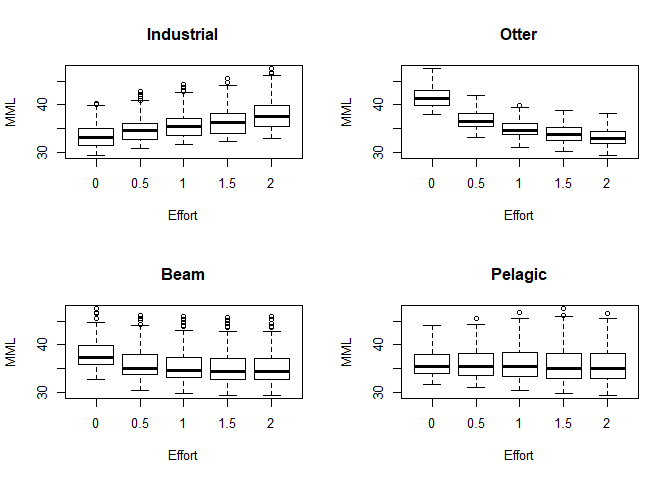All three indicators appear to be mostly driven by the otter gear, which targets larger fish species such as cod and saithe. This suggests that the three indicators are predominantly metrics of the health of the larger species rather than the smaller species.

We also investigate the state of the stocks relative to the virgin SSB:

# SSB of the final years (tonnes)
new_SSB <- do.call(rbind, lapply(lapply(sce, FUN=get_SSB, time_steps=492:501,
inputs=NS_params), colMeans))/1e6
# Relative SSB
rel_SSB <- t(t(new_SSB)/v_SSB)
colnames(rel_SSB) <- NS_params@species_names

Changes in the relative SSB of saithe based on fishing effort is shown below:

par(mfrow=c(2,2))
boxplot(rel_SSB[, "Saithe"]~efs[, 1], main="Industrial", xlab="Effort",
ylab="Relative SSB")
boxplot(rel_SSB[, "Saithe"]~efs[, 2], main="Otter", xlab="Effort",
ylab="Relative SSB")
boxplot(rel_SSB[, "Saithe"]~efs[, 3], main="Beam", xlab="Effort",
ylab="Relative SSB")
boxplot(rel_SSB[, "Saithe"]~efs[, 4], main="Pelagic", xlab="Effort",
ylab="Relative SSB")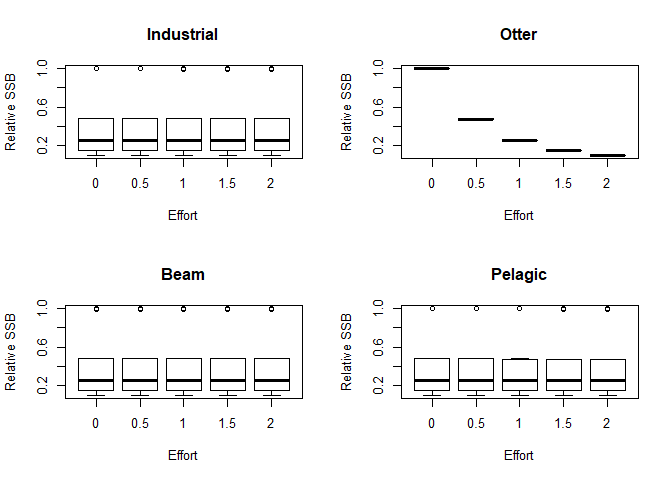As expected, the SSB of saithe is most sensitive to the otter gear, which is the fleet that catches it. We now examine the effects of changes in fishing effort on horse mackerel:

par(mfrow=c(2,2))
boxplot(rel_SSB[, "Horse mackerel"]~efs[, 1], main="Industrial", xlab="Effort",
ylab="Relative SSB")
boxplot(rel_SSB[, "Horse mackerel"]~efs[, 2], main="Otter", xlab="Effort",
ylab="Relative SSB")
boxplot(rel_SSB[, "Horse mackerel"]~efs[, 3], main="Beam", xlab="Effort",
ylab="Relative SSB")
boxplot(rel_SSB[, "Horse mackerel"]~efs[, 4], main="Pelagic", xlab="Effort",
ylab="Relative SSB")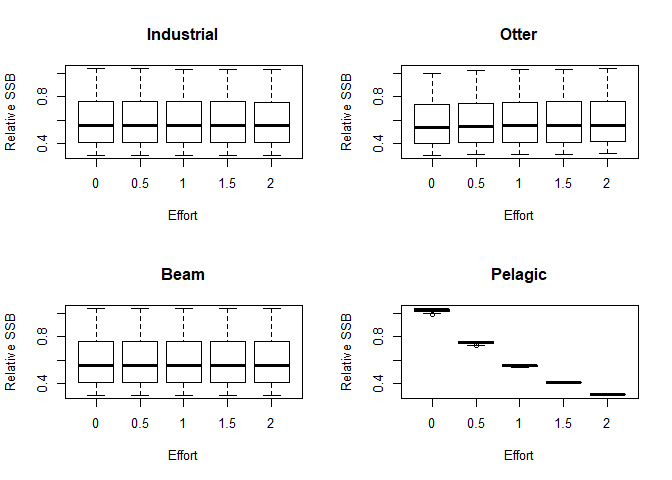The SSB of horse mackerel is most sensitive to the pelagic fleet, which is the only fleet that catches this species. However, horse mackerel SSB is also sensitive to the otter fleet. It is also interesting to note that under zero pelagic fishing, the SSB of horse mackerel can exceed that of the virgin biomass depending on the fishing effort of the other fleets. This is because of predator-prey interactions and the changing dynamics of the other species, particularly a decrease in the predators of horse mackerel.

In Thorpe et al. (2016), a stock is said to be at risk of collapse if the SSB falls below 10% of the virgin SSB. Below we show the number of stocks that are at risk of collapse for each of the fishing scenarios:

risk <- apply(rel_SSB, 1, function(x){return(sum(x<0.1))})

par(mfrow=c(2, 2))
boxplot(risk~efs[, 1], main="Industrial", xlab="Effort", ylab="Stocks at risk")
boxplot(risk~efs[, 2], main="Otter", xlab="Effort", ylab="Stocks at risk")
boxplot(risk~efs[, 3], main="Beam", xlab="Effort", ylab="Stocks at risk")
boxplot(risk~efs[, 4], main="Pelagic", xlab="Effort", ylab="Stocks at risk")

The number of stocks at risk of collapse is sensitive to the otter fleet and the beam fleet. Below we plot the mean number of stocks at risk by varying the effort of the otter and the beam fleets:

z_mat <- outer(ef_lvl, ef_lvl, FUN=function(x, y, efs) {
mapply(function(x, y, efs) {
mean(risk[intersect(which(efs[, 2]==x), which(efs[, 3]==y))])}, x=x, y=y,
MoreArgs=list(efs=efs))
}, efs=efs)
layout(matrix(c(1,1,1,1,1,2), nrow=1, byrow=TRUE))
image(z=-z_mat, x=ef_lvl, y=ef_lvl, axes=T, cex.lab=1.5, xlab="Otter effort", ylab="Beam effort")
axis(1); axis(2); box()
image(z=-matrix(sort(unique(as.numeric(z_mat))), nrow=1),
y=sort(unique(as.numeric(z_mat))), axes=F, cex.lab=1.5,
ylab="Number of stocks at risk")
axis(2); box()
box()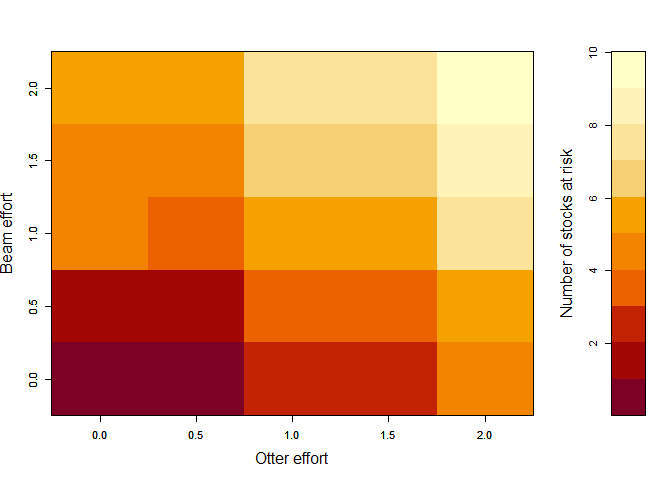## Dynamic fishing

We now investigate a dynamical fishing scenario. We first set up effort for the four gears with varying fishing effort, shown below:

Industrial <- rep(1.5, 20)
Otter <- -1/100*1:20*(1:20-20)
Beam <- 1:20*1/20+0.25
Pelagic <- 1+c(1:5*1/5, 5:1*1/5, 1:5*1/5, 5:1*1/5)
par(mfrow=c(2, 2))
plot(1:20, Industrial, ylab="Effort", main="Industrial", xlab="Year",
ylim=c(0, 2), type="s")
plot(1:20, Otter, ylab="Effort", main="Otter", xlab="Year",
ylim=c(0, 2), type="s")
plot(1:20, Beam, ylab="Effort", main="Beam", xlab="Year",
ylim=c(0, 2), type="s")
plot(1:20, Pelagic, ylab="Effort", main="Pelagic", xlab="Year",
ylim=c(0, 2), type="s")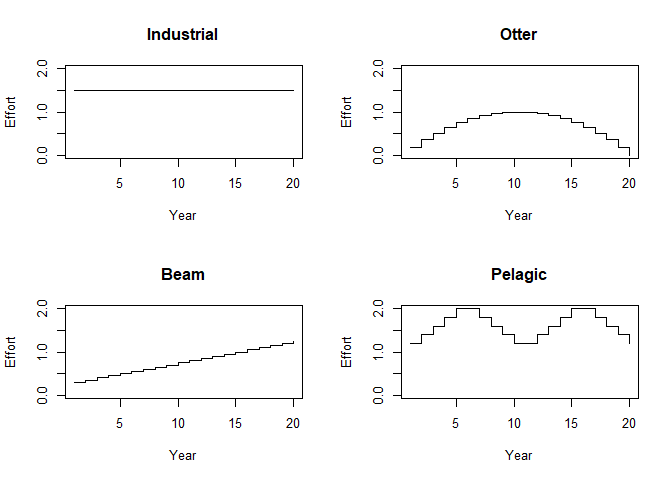We then run the model for 20 years from the unfished state (model_run) and calculate the annual catches for those 20 years:

# Set up effort for the model run
effort_mat <- cbind(Industrial, Otter, Beam, Pelagic)
colnames(effort_mat) <- c("Industrial", "Otter", "Beam", "Pelagic")
model_run_dyn <- run_LeMans(params=NS_params, N0=model_run@N[,,501],
years=20, effort=effort_mat)
catches <- get_annual_catch(inputs=NS_params, outputs=model_run_dyn)/1e6 # in tonnes
colnames(catches) <- NS_params@species_names

We can then plot the annual catches for different species:

par(mfrow=c(2, 2))
plot(1:20, catches[, "Sprat"], type="l", main="Sprat", xlab="Year",
ylab="Catch (tonnes)", ylim=c(0, max(catches[, "Sprat"])))
plot(1:20, catches[, "Cod"], type="l", main="Cod", xlab="Year",
ylab="Catch (tonnes)", ylim=c(0, max(catches[, "Cod"])))
plot(1:20, catches[, "Sole"], type="l", main="Sole", xlab="Year",
ylab="Catch (tonnes)", ylim=c(0,max(catches[, "Sole"])))
plot(1:20, catches[, "Herring"], type="l", main="Herring", xlab="Year",
ylab="Catch (tonnes)", ylim=c(0, max(catches[, "Herring"])))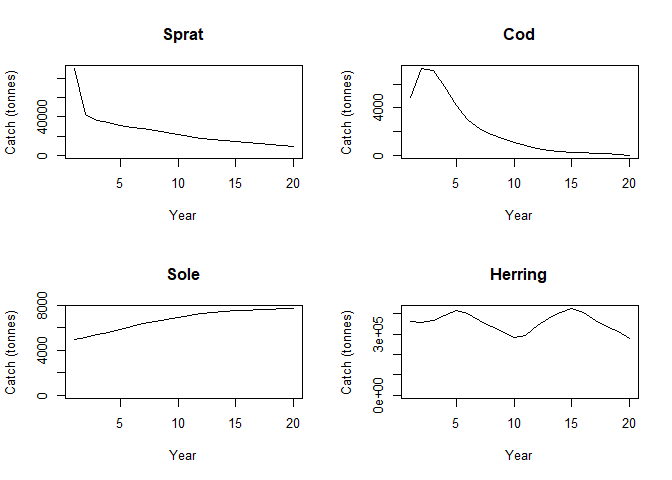We can see that sprat and cod is very quickly overfished. We know this because increases in effort beyond a certain point result in a decrease in the total catch of cod. This occurs as the stock size continually decreases for much of the model run, which we can see below. The catch of sole increases as effort increases and the catch of herring also follows the effort dynamics. Below we plot the SSB (in grams):

plot_SSB(inputs=NS_params, outputs=model_run_dyn,
species=c("Sprat", "Cod", "Sole", "Herring"),
full_plot_only=FALSE)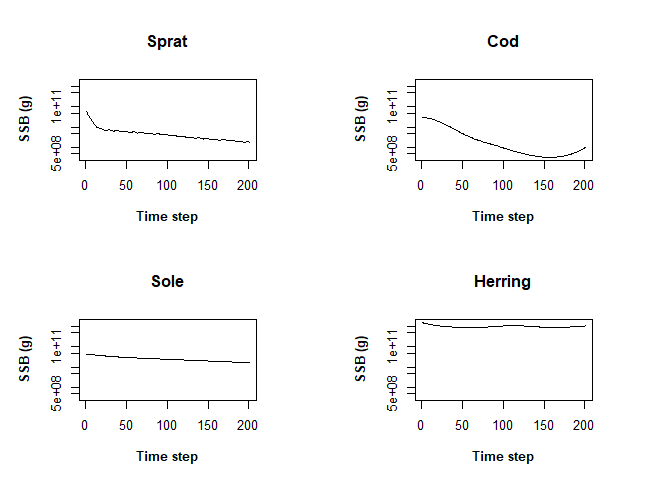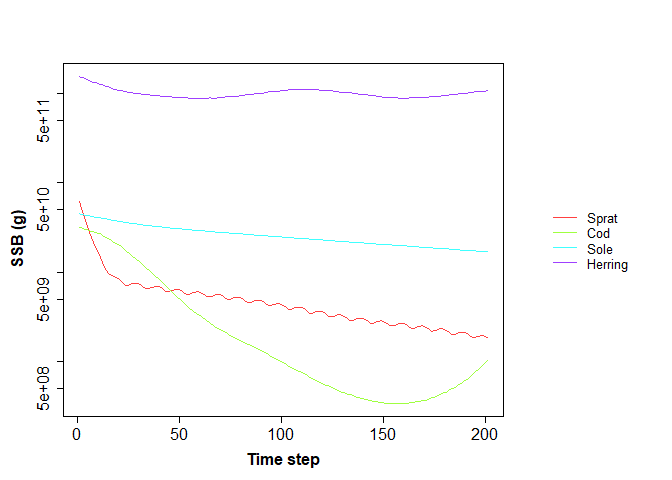We also calculate the total catch for each gear and plot it for each year:

catch_per_gear <- get_CPG(inputs=NS_params, outputs=model_run_dyn, effort=effort_mat)
tot_pg <- apply(catch_per_gear, c(2, 3), sum)/1e6
year <- rep(1:20, each=10)
# Total catch per gear per year
tot_pgpy <- t(sapply(1:20, function(x, year){
tele <- which(year==x)
if (length(tele)>1){
return(rowSums(tot_pg[, tele]))
}
return(tot_pg[, tele])
}, year=year))

par(mfrow=c(2, 2))
plot(1:20, tot_pgpy[, 1], type="l", ylim=c(0, max(tot_pgpy[, 1])), xlab="Year",
main="Industrial", ylab="Catch (tonnes)")
plot(1:20, tot_pgpy[, 2], type="l", ylim=c(0, max(tot_pgpy[, 2])), xlab="Year",
main="Otter", ylab="Catch (tonnes)")
plot(1:20, tot_pgpy[, 3], type="l", ylim=c(0, max(tot_pgpy[, 3])), xlab="Year",
main="Beam", ylab="Catch (tonnes)")
plot(1:20, tot_pgpy[, 4], type="l", ylim=c(0, max(tot_pgpy[, 4])), xlab="Year",
main="Pelagic", ylab="Catch (tonnes)")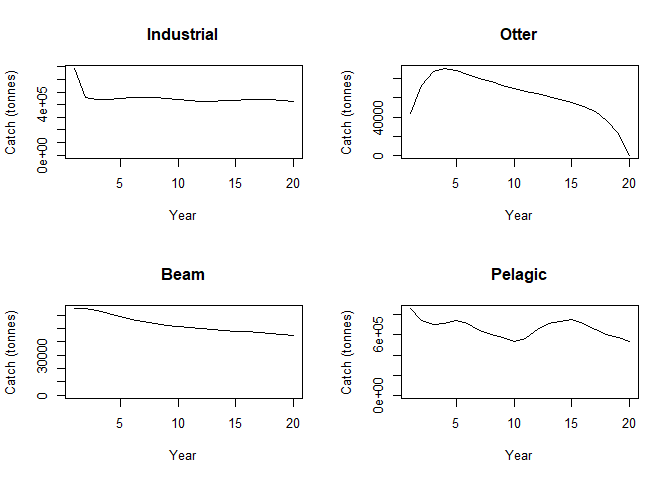We can also investigate the Catch Per Unit Effort (CPUE) for each species. We calculate the mean CPUE across each year:

CPUE <- get_CPUE(inputs=NS_params, outputs=model_run_dyn, effort=effort_mat)/1e6
cpue_py <- t(sapply(1:20, function(x, year){
tele <- which(year==x)
if (length(tele)>1){
return(colMeans(CPUE[tele, ]))
}
return(CPUE[tele, ])
}, year=year))

colnames(cpue_py) <- NS_params@species_names

par(mfrow=c(2, 2))
plot(1:20, cpue_py[, "Sprat"], type="l", main="Sprat", xlab="Year",
ylab="CPUE (tonnes)", ylim=c(0, max(cpue_py[, "Sprat"])))
plot(1:20, cpue_py[, "Cod"], type="l", main="Cod", xlab="Year",
ylab="CPUE (tonnes)", ylim=c(0, max(cpue_py[, "Cod"])))
plot(1:20, cpue_py[, "Sole"], type="l", main="Sole", xlab="Year",
ylab="CPUE (tonnes)", ylim=c(0, max(cpue_py[, "Sole"])))
plot(1:20, cpue_py[, "Herring"], type="l", main="Herring", xlab="Year",
ylab="CPUE (tonnes)", ylim=c(0, max(cpue_py[, "Herring"])))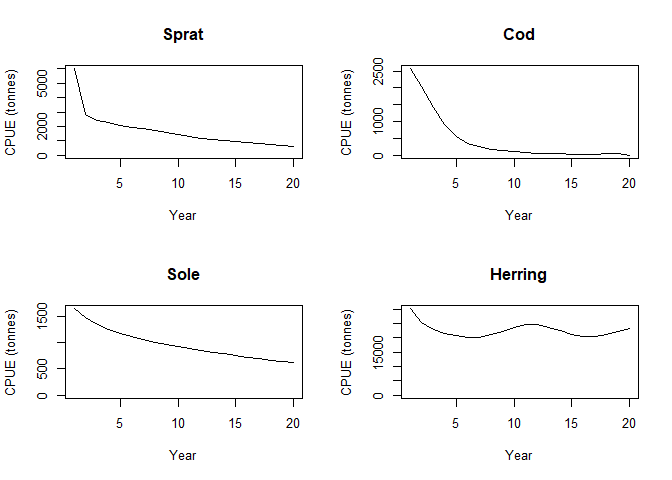Sprat, Cod and sole are always overfished and herring is overfished in some years and underfished in others.

In LeMaRns it is possible for a species to be caught by multiple gears. To demonstrate this we add another fleet - a Recreational fleet. The selectivity for this fleet is knife-edge and it catches cod, haddock, herring, horse mackerel, mackerel, plaice, saithe and whiting, with all fish exceeding the minimum landing size Lmin being retained:

rec_fish <- data.frame(catch_species=c("Cod", "Haddock", "Herring", "Horse mackerel",
"Mackerel", "Plaice", "Saithe", "Whiting"),
curve=rep("knife-edge"), gear_name="Recreational")
rec_fish$max_catchability <- c(0.01, 0.01, 0.005, 0.05, 0.05, 0.01, 0.01, 0.02) Lmin <- c(35, 30, 20, 15, 30, 27, 35, 27) To run the LeMansParam function rec_fish and NS_mixed_fish need to be combined: gdf <- rbind(NS_mixed_fish, rec_fish) and L50, eta and Lmin must be the same size as nrow(gdf): eta1 <- c(NS_eta, rep(0, 8)) L501 <- c(NS_L50, rep(0, 8)) Lmin1 <- c(rep(0, 21), Lmin) We can then create the LeMans_param object and effort: NS_params_rec <- LeMansParam(df=NS_par, gdf = gdf, tau=NS_tau, eta=eta1, L50=L501, other=NS_other, Lmin=Lmin1) #> Warning in .local(df, gdf, ...): The following columns of df do not match any of the species arguments and were therefore added to recruit_params: #> a, b effort_mat1 <- cbind(effort_mat, 0.1+1:20*0.05/20) colnames(effort_mat1) <- "Recreational" with the effort of the recreational fishing fleet being: plot(1:20, effort_mat1[, "Recreational"], xlab="Years", ylab="Effort", type="l", ylim=c(0, 2), main="Recreational")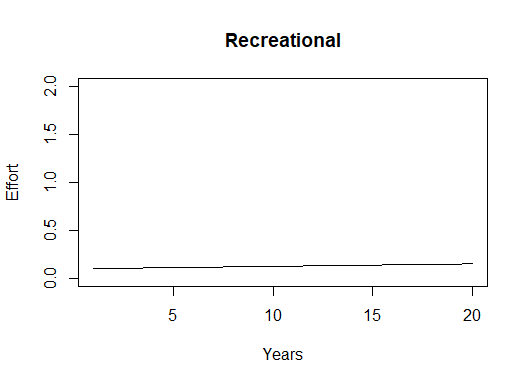We then run the model: model_run_rec <- run_LeMans(params=NS_params_rec, N0=model_run@N[,,501], years=20, effort=effort_mat1) and explore the catches for the recreational fleet: catch_per_gear_rec <- get_CPG(inputs=NS_params_rec, outputs=model_run_rec, effort=effort_mat1) rec_py <- t(sapply(1:20, function(x, year){ tele <- which(year==x) if (length(tele)>1){ return(rowSums(catch_per_gear_rec[, 5, tele])) } return(catch_per_gear_rec[, 5, tele]) }, year=year))/1e6 colnames(rec_py) <- NS_params_rec@species_names par(mfrow=c(2, 2)) plot(1:20, rec_py[, "Herring"], type="l", main="Herring", xlab="Year", ylab="Catch (tonnes)") plot(1:20, rec_py[, "Mackerel"], type="l", main="Horse mackerel", xlab="Year", ylab="Catch (tonnes)") plot(1:20, rec_py[, "Cod"], type="l", main="Cod", xlab="Year", ylab="Catch (tonnes)") plot(1:20 ,rec_py[, "Saithe"], type="l", main="Saithe", xlab="Year", ylab="Catch (tonnes)")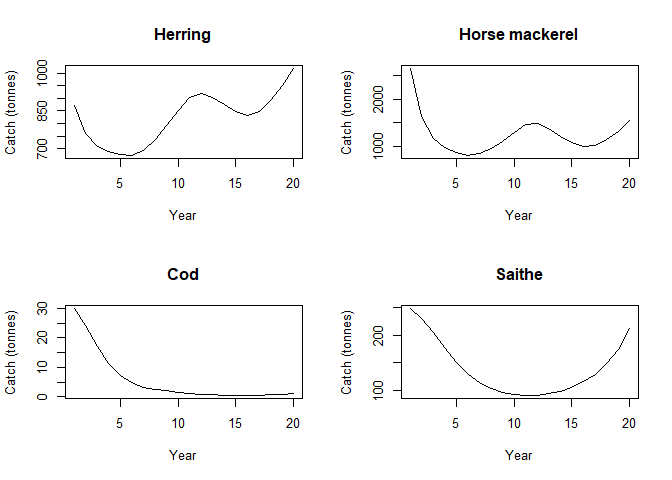## Nash equilibrium Fish stocks are often managed by considering the fishing mortality that maximises the long-term yield, i.e. the Maximum Sustainable Yield (MSY). We can define $$f_i(F_i, F_{-i})$$ as the long-term catch of the $$i$$th stock, where $$F_i$$ is the fishing mortality of the $$i$$th stock and $$F_{-i}$$ is the fishing mortalities of the other stocks. Many stocks are managed on a stock by stock basis using single-species models. This means that $\begin{equation*} f_i(F_i,F_{-i})=f_i(F_i,F'_{-i}), \end{equation*}$ $$\forall{}F'_{-i}$$ and we can define $\begin{equation*} F_{MSY,i}={\text{arg}\max}_{F_i}f_i(F_i,F'_{-i}). \end{equation*}$ In the single-species world this is commonly well defined. However, in the multispecies world stocks interact with one another and the fishing mortality of the $$j$$th stock affects the catch of the $$i$$th stock, i.e. $\begin{equation*} f_i(F_i,F_{-i})\neq{}f_i(F_i). \end{equation*}$ We therefore need to define a multispecies maximum sustainable yield. One possibility is the Nash equilibrium Thorpe et al. (2016), which is defined as the point at which we are unable to increase $$f_i(F_i,F_{-i})$$ by changing $$F_i$$ only, for all $$i$$. Formally, $$F_{Nash,i},\forall{}i$$ is a Nash equilibrium when $\begin{equation*} \forall{}i,F_i:f_i(F_{Nash,i},F_{Nash,-i})\geq{}f_i(F_{i},F_{Nash,-i}). \end{equation*}$ We use the LeMaRns package to calculate the Nash equilibrium. First we initialise a model run: NS_params_n <- LeMansParam(NS_par, tau=NS_tau, eta=NS_eta, L50=NS_L50, other=NS_other) #> Warning in .local(df, gdf, ...): The following columns of df do not match any of the species arguments and were therefore added to recruit_params: #> a, b model_run_n_init <- run_LeMans(NS_params_n, years=50) # Set the initial state N0 <- model_run_n_init@N[,,501] We then create a function that returns the negative mean catch of the ith species in the 20th year with fishing effort x for the ith species and eff for the rest. As we have set apply(NS_params_n@Qs,3,max) to be 1 using max_catchability, the maximum fishing mortality of each species is eff. calc_catch<-function(x,i,eff){ eff[i] <- x tmp <- run_LeMans(NS_params_n, N0=N0, years=20, effort=matrix(eff, nrow=20, ncol=21, byrow=T)) return(mean(tail(colSums(tmp@Catch[,i,]), 10))) } Starting from $$F_{MSY}$$ in Thorpe et al. (2016), we iteratively optimise the fishing effort for each species. We continue this process until the difference in the optimal effort and the effort at the start of the optimisation differ by less than 0.01 for all species. eff <- fmsy <-c(1.3, 0.35, 0.35, 0.72, 0.6, 0.41, 0.25, 0.5, 0.33, 0.22, 0.32, 0.21, 0.27, 0.27, 0.25, 0.15, 0.30, 0.11, 0.1, 0.19, 0.3) stat <- rep(FALSE, 21) while(any(stat==FALSE)){ for (i in 1:21){ opts <- optim(eff[i], calc_catch, i=i, eff=eff, method="Brent", lower=0, upper=2,control = list(fnscale = -1)) stat[i] <- abs(eff[i]-opts$par)<0.01
eff[i] <- opts$par } } eff is the Nash equilibrium. Below we plot the SSB for the Nash equilibrium: nash <- run_LeMans(NS_params_n, N0=N0, years=20, effort=matrix(eff, nrow=20, ncol=21, byrow=T)) plot_SSB(inputs=NS_params_n, outputs=nash)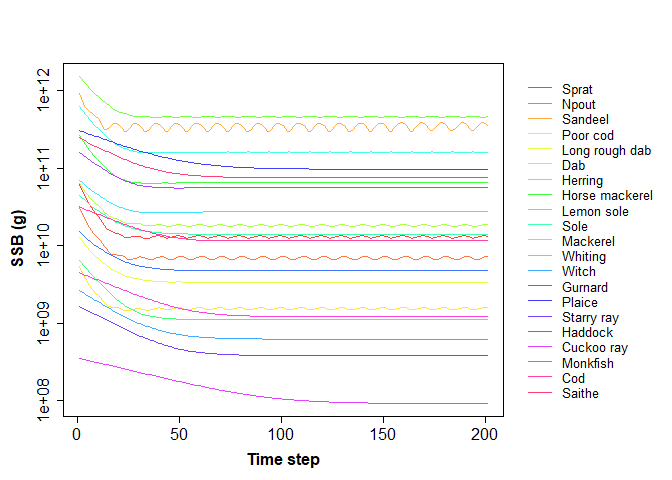To compare this to the single-species MSY we have to change the rules slightly as we have to specify the fishing mortality rates for the other species. Arbitrarily we choose to set effort=fmsy for all the but the $$i$$th species when calculating $$F_{MSY,i}$$. This is: fmsy_lm <- sapply(1:21, function(i, eff){optim(eff[i], calc_catch, i=i, eff=eff, method="Brent", lower=0, upper=2,control = list(fnscale = -1))$par},
eff=fmsy)
msy <- run_LeMans(NS_params_n, N0=N0, years=20, effort=matrix(fmsy_lm, nrow=20, ncol=21, byrow=T))

We then compare $$F_{MSY}$$ and $$F_{Nash}$$ in this study:

plot(fmsy_lm, eff, ylab=expression(F[Nash]), xlab=expression(F[MSY]))
abline(a=0, b=1)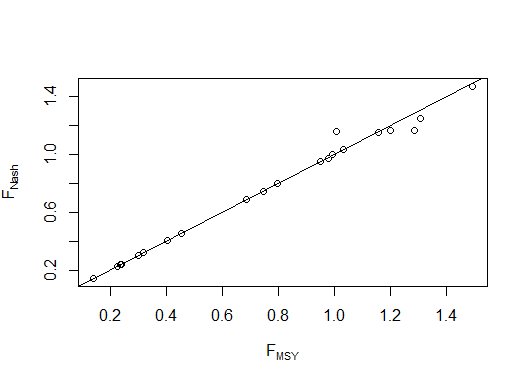The two appear to be similar for most species, particularly those with lower values of $$F_{MSY}$$ and $$F_{Nash}$$, however they appear to differ more for larger values.

We comment on the arbitrariness of the choice of fishing mortality by pointing out that had we set the other species fishing mortality values to be $$F_{Nash,-i}$$ when calculating $$F_{MSY,i}$$, then $$F_{MSY,i}=F_{Nash,i}$$ by definition.